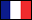﻿ WinSTAT Statistics Software Add-In for Microsoft Excelthe Statistics Add-In for Microsoft® Excel## Welcome!(français)(deutsch)

WinSTAT is the statistics Add-In for Microsoft Excel, and this is the place to find out all about it.

Tired of your hard-to-use, need-to-be-a-fulltime-expert statistics software? Find out why WinSTAT is the software for you.

Wondering if WinSTAT covers the functions and graphics you need? Let the function reference page surprise you, complete with sample outputs of tables and graphics for all functions.

Still not convinced? There's no way to be sure until you've tried WinSTAT for yourself. We've got the demo download right here.

We're certain that WinSTAT is in your future. Why not make the future happen? Order WinSTAT now.

Some important functions at a glance:

• Regression analysis: linear regression, multiple regression, polynomial regression
• Correlation: Pearson correlation, Spearman correlation, partial correlation
• Analysis of variance (ANOVA)
• Repeated measures ANOVA
• Survival analysis (Kaplan-Meier)
• Cox regression
• Discriminant analysis
• Cluster analysis
• Factor analysis
• t-Tests: dependent t-test, independent t-test
• Mann-Whitney U-test
• Wilcoxon test
• Kruskal-Wallis H-test
• Box-plot, scatterplot, histogram, probit chart
• Crosstabs
• Fisher's exact test
• Kolmogorov-Smirnov, Shapiro-Wilk, Chi-square test
• McNemar
• Tests of normal distribution
• Tests of randomness
• Outlier tests
• Pareto diagram, Quality control charts, Process capability

All functions for one price: \$99 per license.

WinSTAT is a product of:

E-Mail: